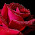# Hexadecimal numbers to octal numbers

Convert hexadecimal numbers to octal numbers
1. F4
3. 8C1F

Completion:
1. F4 (16) = ? (8)
* First, convert hexadecimal numbers to decimal numbers. Then, convert decimal numbers to octal numbers.

F4 = 4x160 + Fx161
F4 = 4x160 + 15x161
F4 = 4 + 240
F4 (16) = 244 (10)

decimal to octal
244 / 8 = 30, remains 4
30 / 8 = 3, remains 6
3 / 8 = 0, remains 3
244 (10) = 364 (8)

F4 hexadecimal number equal to 364 octal number
F4 (16) = 364 (8)

2. ADE (16) = ? (8)
ADE = Ex160 + Dx161 + Ax162
ADE = 14x160 + 13x161 + 10x162
ADE = 14 + 208 + 2560

decimal to octal
2782 / 8 = 347, remains 6
347 / 8 = 43, remains 3
43 / 8 = 5, remains 3
5 / 8 = 0, remains 5
3501 (10) = 5336 (8)

3. 8C1F (16) = ? (8)
8C1F = Fx160 + 1x161 + Cx162 + 8x163
8C1F = 15x160 + 1x161 + 12x162 + 8x163
8C1F = 15 + 16 + 3072 + 32768
8C1F (16) = 35871 (10)

decimal to octal
35871 / 8 = 4483, remains 7
4483 / 8 = 560, remains 3
560 / 8 = 70, remains 0
70 / 8 = 8, remains 6
8 / 8 = 1, remains 0
1 / 8 = 0, remains 1
605025 (10) = 106037 (8)

8C1F hexadecimal number equal to 106037 octal number
8C1F (16) = 106037 (8)

Next→
←Prev

### Choose label

1.2.